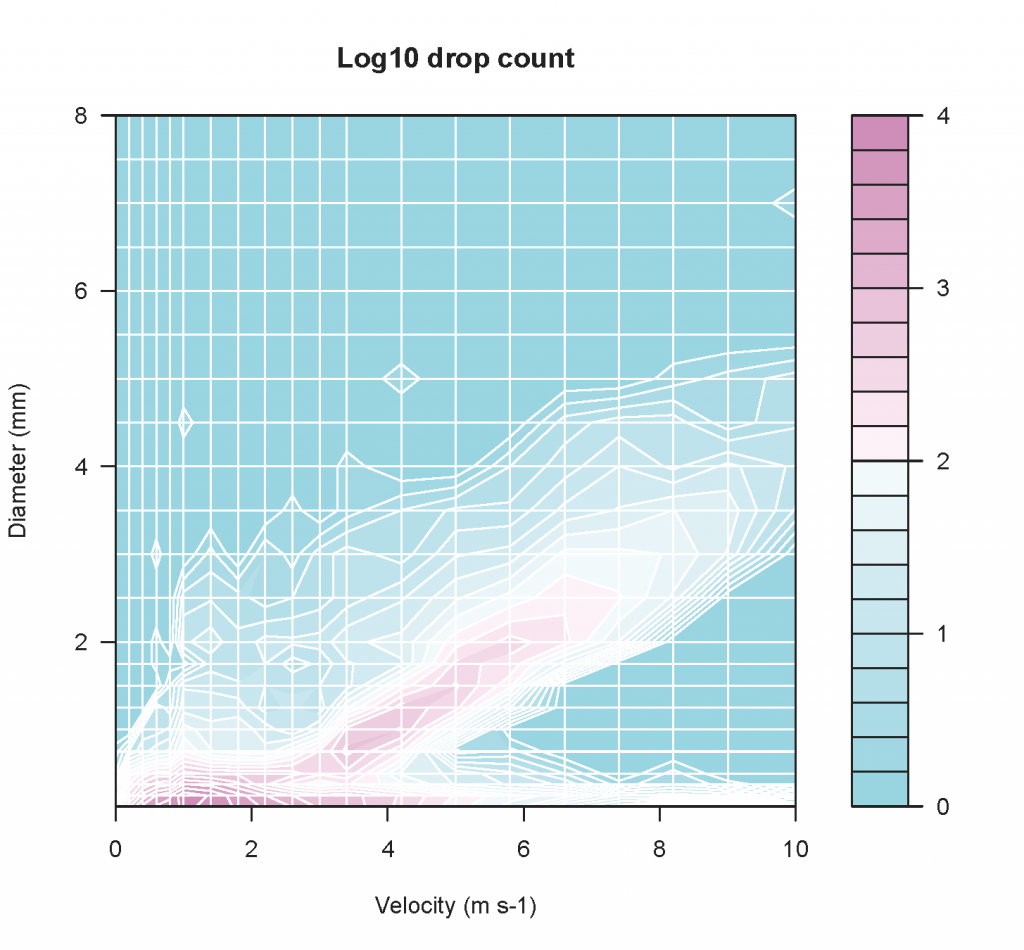## Uses of rainfall spectrum data

The scientific interest in rainfall spectrum (its drop size distribution, DSD) is double. On one hand it provides information about cloud physics and rainfall generating processes. On the other hand, the DSD controls relevant rainfall properties such as its kinetic energy (relevant for soil erosion studies), the reflectivity of microwaves (relevant for the remote sensing of precipitation) or the optical attenuation of visible light.

Optical disdrometers or pluvioespectrometers are devices that register the diameter and fall velocity of raindrops from the attenuation they produce on an infrared laser beam. Current devices classify the falling drops into a number of size and velocity classes or bins, and count their number over a given time interval. The plot in Figure 1 is an example rainfall spectrum as registered by our disdrometer in Aula Dei. White straight lines show the discrete data bins of the device (22 diameter and 20 velocity classes). The isolines have been interpolated from the original drop count data.Figure 1. Rainfall spectrum: number of drops as a function of their size and fall velocity, event of 2010-03-24 18:56 to 2010-03-24 19:42 GMT, Aula Dei disdrometer. Note the logarithmic scale.

Integral rain parameters can be derived from DSD data according to the general expression:

where is a constant independent of the drop size, is a parameter that varies with the drop size, and and is the number of drops per unit volume with diameters between D and .

Since the disdrometer quantizes the DSD into discrete bins, the above integral is computed as a sum over the drop size classes. The expressions for the drop number density N (), rain rate R (), liquid water content M (), reflectivity in Rayleigh scattering regime Z (), kinetic energy E () and MOR visibility V (contrast 5%, )are (Tokay et al., 2001):

where is the disdrometer sampling cross section (), is the sampling time interval (), is the density of water (), is the drop number count for disdrometer channel , and and are the corresponding median raindrop size () and velocity ().